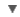POS
WORDNET DICTIONARY
CIDE DICTIONARY
OXFORD DICTIONARY
Link, Gadget and Share#gauss

RELATED WORDS :

:
Noun

## CIDE DICTIONARY

gaussn. [So named after Karl F. Gauss, a German mathematician.].
•  The C.G.S. unit of density of magnetic field, equal to a field of one line of force per square centimeter, being thus adopted as an international unit at Paris in 1900; sometimes used as a unit of intensity of magnetic field. It was previously suggested as a unit of magnetomotive force.  [Webster 1913 Suppl.]
•  Karl F. Gauss, a German mathematician.  [PJC]

## OXFORD DICTIONARY

gauss, n. (pl. same or gausses) a unit of magnetic induction, equal to one ten-thousandth of a tesla.

Usage
Abbr.: G.
Etymology
K. Gauss, Ger. mathematician d. 1855
copyright © 2012 Yayasan Lembaga SABDA (YLSA) | To report a problem/suggestion